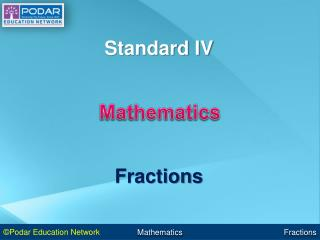DownloadDownload PresentationMathematics

# Mathematics

Télécharger la présentation## Mathematics

- - - - - - - - - - - - - - - - - - - - - - - - - - - E N D - - - - - - - - - - - - - - - - - - - - - - - - - - -
##### Presentation Transcript

1. Mathematics Fractions

2. Terminology Like decimals, fractions represent parts of numbers. A fraction is usually a number that is between 0 and 1. Numerator - number on top Denominator - number on bottom

3. To add or subtract fractions with like denominators: • If the denominators are already the same, then you’re ready to add or subtract. • Add or subtract the numerators. Keep the denominators the same. • Simplify (reduce) the fraction, if possible. • If you end up with an improper fraction, change it to a mixed number.

4. Examples 1 2 3 + = 5 5 5 This answer cannot be reduced any further.

5. 9 4 5 2 2 = ÷ = 14 14 14 7 The GCF of 4 and 14 is 2, so we divide by 2 to reduce.

6. 8 3 11 + = 9 9 9 This is an improper fraction (the top is bigger than the bottom), so we want to change it to a mixed number. To do this, divide the bottom into the top. 1 Answer: 1 2 11 9 9 9 2

7. Try Some 7 5 1 = 6 12 12 4 1 5 + = 7 7 7 1 12 1 8 + = 15 3 15